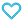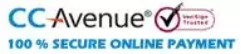# MTG Vedic Mathematics Vol-2 : Multiplication

99.00

Discount offer on this book in a bundle, click to view

Vedic Mathematics is a collection of Techniques/Sutras to solve mathematical calculation in easy and faster way. It consists of 16 Sutras and Upsutras. It represents step by step process to solve the problems followed by illustrations for skilful learning. The series comprises of 7 volumes that Sharpens your mind, increases mental agility and intelligence.

338 in stock

Qty:Add to WishlistVedic Maths Course is for students, teachers, mentors, homemakers and even businessmen for doing calculations more efficiently. With the help  of Vedic Maths, one can perform the calculations 10-15 times faster than the conventional maths methods.

• 1. Introduction to Multiplication (By Vedic Sutra)
• 2. Multiplication of Two Numbers (When both the numbers are below the base 10)
• 3. Multiplication of Two Numbers (When both the numbers are below the base 100)
• 4. Multiplication of Two Numbers (When both the numbers are below the base 1000)
• 5. Multiplication of Two Numbers (When both the numbers are above the base 10)
• 6. Multiplication of Two Numbers (When both the numbers are above the base 100)
• 7. Multiplication of Two Numbers (When both the numbers are above the base 1000)
• 8. Multiplication of Two Numbers (When one number is above and the other is below the base 10)
• 9. Multiplication of Two Numbers (When one number is above and the other is below the base 100)
• 10. Multiplication of Two Numbers (When one number is above and the other is below the base 1000)
ISBN11 9789391191481 MTG Editorial Board 2020-21 56 Others Others Mathematics 100gm

## Reviews

There are no reviews yet.

## Customers prefer to buy this combination offer...

• ### MTG Vedic Mathematics combo Vol-1 to 7

Total Price = 693.00

Combo Price = 519.75